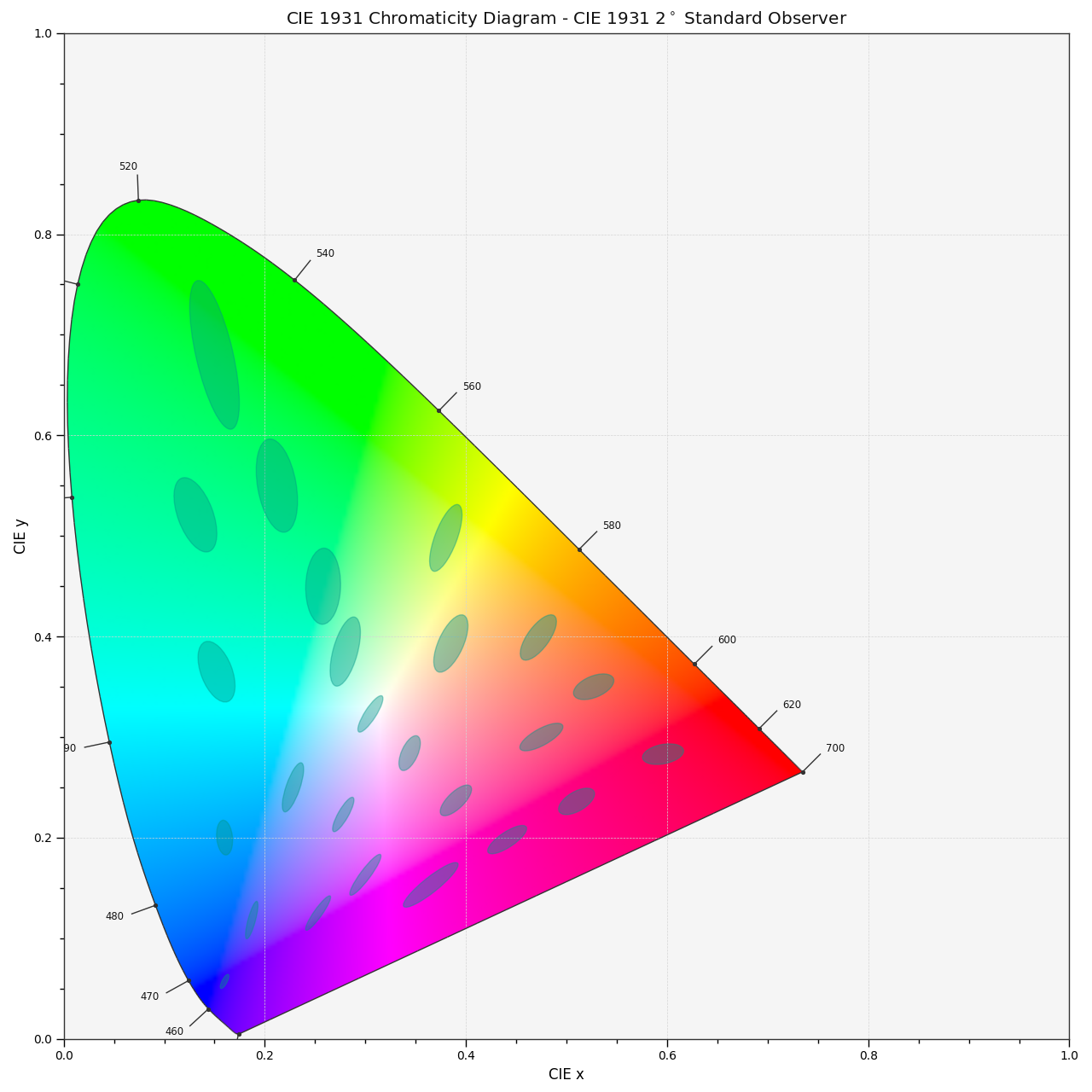colour.plotting.plot_ellipses_MacAdam1942_in_chromaticity_diagram_CIE1931(chromaticity_diagram_callable_CIE1931=<function plot_chromaticity_diagram_CIE1931>, chromaticity_diagram_clipping=False, ellipse_parameters=None, **kwargs)[source]

Plots MacAdam (1942) Ellipses (Observer PGN) in the CIE 1931 Chromaticity Diagram.

Parameters
• chromaticity_diagram_callable_CIE1931 (callable, optional) – Callable responsible for drawing the CIE 1931 Chromaticity Diagram.

• chromaticity_diagram_clipping (bool, optional,) – Whether to clip the CIE 1931 Chromaticity Diagram colours with the ellipses.

• ellipse_parameters (dict or array_like, optional) – Parameters for the Ellipse class, ellipse_parameters can be either a single dictionary applied to all the ellipses with same settings or a sequence of dictionaries with different settings for each ellipse.

Other Parameters

**kwargs (dict, optional) – {colour.plotting.artist(), colour.plotting.diagrams.plot_chromaticity_diagram(), colour.plotting.models.plot_ellipses_MacAdam1942_in_chromaticity_diagram()}, colour.plotting.render()}, Please refer to the documentation of the previously listed definitions.

Returns

Current figure and axes.

Return type

tuple

Examples

>>> plot_ellipses_MacAdam1942_in_chromaticity_diagram_CIE1931()
...
(<Figure size ... with 1 Axes>, <matplotlib.axes._subplots.AxesSubplot object at 0x...>)